Test: Discrete Time Signals

# Test: Discrete Time Signals

Test Description

## 15 Questions MCQ Test Signals and Systems | Test: Discrete Time Signals

Test: Discrete Time Signals for Electronics and Communication Engineering (ECE) 2023 is part of Signals and Systems preparation. The Test: Discrete Time Signals questions and answers have been prepared according to the Electronics and Communication Engineering (ECE) exam syllabus.The Test: Discrete Time Signals MCQs are made for Electronics and Communication Engineering (ECE) 2023 Exam. Find important definitions, questions, notes, meanings, examples, exercises, MCQs and online tests for Test: Discrete Time Signals below.
Solutions of Test: Discrete Time Signals questions in English are available as part of our Signals and Systems for Electronics and Communication Engineering (ECE) & Test: Discrete Time Signals solutions in Hindi for Signals and Systems course. Download more important topics, notes, lectures and mock test series for Electronics and Communication Engineering (ECE) Exam by signing up for free. Attempt Test: Discrete Time Signals | 15 questions in 45 minutes | Mock test for Electronics and Communication Engineering (ECE) preparation | Free important questions MCQ to study Signals and Systems for Electronics and Communication Engineering (ECE) Exam | Download free PDF with solutions
 1 Crore+ students have signed up on EduRev. Have you?
Test: Discrete Time Signals - Question 1

### Which of the following is NOT one of the representations of discrete-time signals?

Detailed Solution for Test: Discrete Time Signals - Question 1

1) Functional Representation.

Example: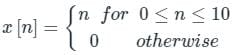2) Tabular method of representation

Example: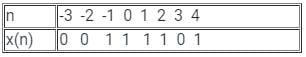3) Sequence Representation

Example: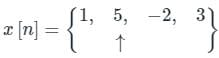​​​​​​​

Test: Discrete Time Signals - Question 2

### Which type/s of discrete-time system do/does not exhibit the necessity of any feedback?

Detailed Solution for Test: Discrete Time Signals - Question 2

Recursive discrete time system:

If a system output y(n) at time n depends on any number of past output value y(n – 1), y(n – 2), …, it is called a recursive system.

This system requires two multiplication, one addition, and one memory location. This is a recursive system which means the output at time n depends on any number of a past output values.

So, a recursive system has feedback output of the system into the input. This feed back loop contains a delay element.

Non-Recursive discrete time system:

If y(n) depends only on the present and past input, it is called non-recursive. It does not exhibit the necessity of any feedback.

Test: Discrete Time Signals - Question 3

### The convolution y(n) = (-0.5)n * 2n u(2n + 2) is

Detailed Solution for Test: Discrete Time Signals - Question 3

Concept:

Output = Input × Eigen value.

Calculation:

Let, Input x(n) =  (-0.5)n

h(n) = 2n u(2n + 2)

= 2n u(n + 1)

= 2n+1 u(n+1) / 2

(scaling does not affect the discrete-time signal)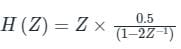putting -0.5 in place of Z, we get: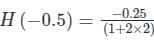= -1/20

-1/20 is the eigenvalue

Output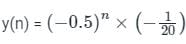Test: Discrete Time Signals - Question 4

Express the following finite discrete-time signal as the difference of two unit step sequences: x[n] = 1, for 0 ≤ n ≤ 5; and 0 otherwise.

Detailed Solution for Test: Discrete Time Signals - Question 4

Concept:

The signal u[n] is defined as:

u[n] = 1 for n ≥ 0

u[n] = 0 for n < 0

Also, the shifted version of u[n] is defined as:

u[n - n0] = 1 for n ≥ n0

u[n - n0] = 0 for n < n0

Now the combination of two discrete time signals can be analysed as:

u[n] - u[n - n0] = 1 for 0 ≤ n ≤  n0 - 1

u[n] - u[n - n0] = 0, otherwise

Application:

Since the given signal is defined as:

x[n] = 1, for 0 ≤ n ≤ 5

We can write:

x[n] = u[n] - u[n - 6]

Test: Discrete Time Signals - Question 5

The discrete time system described by y(n) = [x(n)]2 is

Detailed Solution for Test: Discrete Time Signals - Question 5

Concept:

Linearity: Necessary and sufficient condition to prove the linearity of the system is that the linear system follows the laws of superposition i.e. the response of the system is the sum of the responses obtained from each input considered separately.

y{ax1[t] + bx2[t]} = a y{x1[t]} + b y{x2[t]}

Conditions to check whether the system is linear or not.

• The output should be zero for zero input
• There should not be any non-linear operator present in the system.

Causal system:

If O/P of the system is independent of the future value of input then the system is said to be causal. Causal systems are practical or physically reliable systems.

Analysis:

y(n) = [x(n)]2

Linearity check:

x1(n) → x1(n)2

x2(n) → x2(n)2

x3(n) = [x1(n) + x2(n)] →  [x1(n) + x2(n)]2 = x1(n)2 + x2(n)2 + 2x1(n) x2(n)

≠ x1(n)2 + x2(n)2

∴ Non - linear

Causality check:

y(0) = x(0)2

y(1) = x(1)2

y(-1) = x(-1)2

∴ the system is causal.

Test: Discrete Time Signals - Question 6

The special case of a finite-duration sequence is given as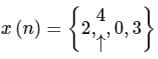The sequence x(n) into a sum of weighted impulse sequences will be

Detailed Solution for Test: Discrete Time Signals - Question 6The arrow indicates the origin. This can be represented as: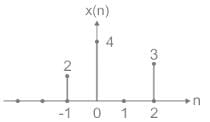In terms of unit impulse sequences, this can be represented as:

x(n) = 2δ(n + 1) + 4δ(n) + 3δ(n – 2)

Test: Discrete Time Signals - Question 7

What is the nature of the following function: y[n] = y[n-1] + x[n]?

Detailed Solution for Test: Discrete Time Signals - Question 7

If the above recursive definition is repeated for all n, starting from 1,2.. then y[n] will be the sum of all x[n] ranging from 1 to n, making it an accumulator system.

Test: Discrete Time Signals - Question 8

Is the function y[n] = x[n - 1] – x[n - 56] causal?

Detailed Solution for Test: Discrete Time Signals - Question 8

As the value of the function depends solely on the value of the input at a time presently and/or in the past, it is a causal system.

Test: Discrete Time Signals - Question 9

If n tends to infinity, is the accumulator function a stable one?

Detailed Solution for Test: Discrete Time Signals - Question 9

The system would be unstable, as the output will grow out of bound at the maximally worst possible case.

Test: Discrete Time Signals - Question 10

Discrete-time signals are ________

Detailed Solution for Test: Discrete Time Signals - Question 10

A discrete-time signal is continuous in amplitude and discrete in time. It can either be present in nature or is sampled from an analog signal. A digital signal is discrete in amplitude and time.

Test: Discrete Time Signals - Question 11

Determine the product of two signals: x1 (n) = {2, 1, 1.5, 3}; x2 (n) = { 1, 1.5, 0, 2}.

Detailed Solution for Test: Discrete Time Signals - Question 11

Product of discrete-time signals is computed element by element.
⇒ x(n) = x1 (n) * x2 (n) = {2×1, 1×1.5, 1.5×0, 3×2} = {2,1.5,0,6}.

Test: Discrete Time Signals - Question 12

Is the function y[n] = y[n - 1] + x[n] stable in nature?

Detailed Solution for Test: Discrete Time Signals - Question 12

It is BIBO stable in nature, i.e. bounded input-bounded output stable.

Test: Discrete Time Signals - Question 13

Determine the discrete-time signal: x(n) = 1 for n ≥ 0 and x(n) = 0 for n < 0

Detailed Solution for Test: Discrete Time Signals - Question 13

Unit step is defined by: x(n) = 1 for n ≥ 0 and x(n) = 0 for n < 0.

Test: Discrete Time Signals - Question 14

Determine the value of the summation: ∑n= -∞ δ(n + 3)(n+ n).

Detailed Solution for Test: Discrete Time Signals - Question 14

n= -∞ δ(n + 3)(n+ n)
⇒ δ(n + 3)=1 when n= -3 otherwise 0.
Therefore, the limit reduces to n = -3
⇒ ∑n = -∞ δ(n + 3)(n+ n) = (n+ n)|n = -3 = (-3)2-3 = 9 – 3 = 6.

Test: Discrete Time Signals - Question 15

Unit Impulse function is obtained by using the limiting process on which among the following functions?

Detailed Solution for Test: Discrete Time Signals - Question 15

Unit impulse function can be obtained by using a limiting process on the rectangular pulse function. Area under the rectangular pulse is equal to unity.

## Signals and Systems

40 videos|44 docs|33 tests
 Use Code STAYHOME200 and get INR 200 additional OFF Use Coupon Code
Information about Test: Discrete Time Signals Page
In this test you can find the Exam questions for Test: Discrete Time Signals solved & explained in the simplest way possible. Besides giving Questions and answers for Test: Discrete Time Signals, EduRev gives you an ample number of Online tests for practice

## Signals and Systems

40 videos|44 docs|33 tests